##### Actions

(diff) ← Older revision | Latest revision (diff) | Newer revision → (diff)

A square matrix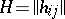of order, with entries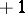or, such that the equation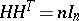(*)

holds, where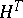is the transposed matrix ofand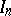is the unit matrix of order. Equality (*) is equivalent to saying that any two rows ofare orthogonal. Hadamard matrices have been named after J. Hadamard who showed  that the determinant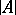of a matrix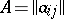of order, with complex entries, satisfies the Hadamard inequality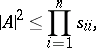where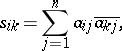and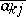is the element conjugate to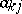(cf. Hadamard theorem on determinants). In particular, if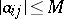, then. It follows that a Hadamard matrix is a square matrix consisting of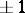's with maximal absolute valueof the determinant. The properties of Hadamard matrices are: 1)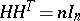implies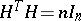and vice versa; 2) transposition of rows or columns and multiplication of the elements of an arbitrary row or column by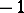again yields a Hadamard matrix; 3) the tensor product of two Hadamard matrices is also a Hadamard matrix, of order equal to the product of the orders of the factors. In other words, ifand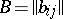are Hadamard matrices of ordersandrespectively, then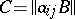is a Hadamard matrix of order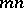. A Hadamard matrix with its first row and first column consisting only ofterms is said to be normalized. The order of a Hadamard matrix is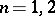or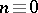(). The normalized Hadamard matrices of orders 1 and 2 are:The existence of a Hadamard matrix has been demonstrated for several classes of values of(see, for example, , ). At the time of writing (the 1980s), it has not yet been proved that a Hadamard matrix exists for any(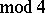). For methods of constructing Hadamard matrices see . Hadamard matrices are used in the construction of certain types of block designs  and codes  (cf. Block design; Code). A Hadamard matrix of order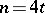is equivalent to a-design.

A generalized Hadamard matrix is a square matrix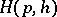of order, with as entries-th roots of unity, which satisfies the equality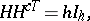whereis the conjugate transpose of the matrixandis the unit matrix of order. Generalized Hadamard matrices have properties analogous to 1) and 3) (cf. ).

How to Cite This Entry: